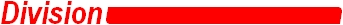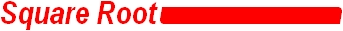“Generating a Benchmark Set of Extremal Rounding Boundary Instances for IEEE Standard Floating Point Division”.

single precision directed rounding

single precision round to nearest

RN.cc

RN64.cc

extremal square root test suite for directed rounding

D.W. Matula HomePage

#The extremal rounding test sets for division, RDp and RNp, provide inputs whose infinitely precise quotients are extremely close to a p-bit number for RDp or a midpoint between 2 p-bit numbers for RNp. These sets provide precisely those inputs whose outputs have the maximum number of like bits after the round bit. Our test set for single precision directed rounding, RD24 and single precision round to nearest, RN24 each contain roughly 5.6 million examples. These examples are sorted, in ASCII format, and may be downloaded in a compressed form.

The C++ program for 32-bit native machines RN.cc and 64-bit native machines RN64.cc will generate the RNi suites for 3 ≤ i ≤ 28, and is further described along with the theory in “Generating a Benchmark Set of Extremal Rounding Boundary Instances for IEEE Standard Floating Point Division”. The count output from the program can be checked with the results in Table 3 of the paper.

#Unlike division, only one square root example for each precision has the maximum number of like bits after the round bit making it extremely close to a p-bit number or a midpoint between 2 p-bit numbers.  Consequently, our single precision extremal square root test suite for directed rounding and our extremal square root test suite for round to nearest contain those inputs whose outputs have at least 12 like bits after the round bit. These suites are in a 2-column ASCII format. The first column is the input for the square root function. The second column indicates the number of like bits after the round bit found in the infinitely precise output.

_______________________________

The contents of these web pages are the sole responsibility of Dr. David W. Matula (matula@engr.smu.edu)

and do not necessarily represent the opinions or policies of Southern Methodist University.

The administrator of this site is Dr. David W. Matula. He may be contacted at matula@engr.smu.edu